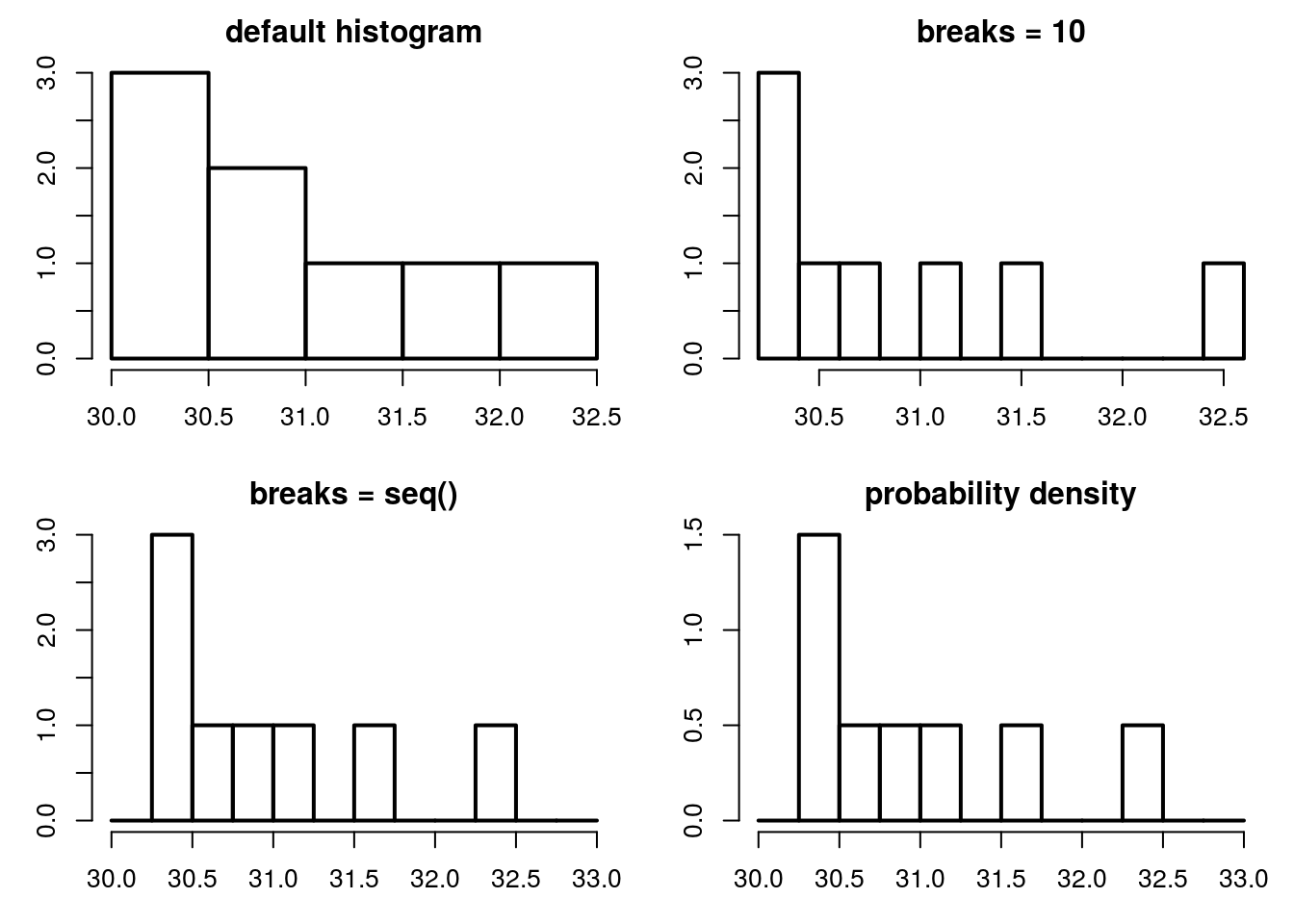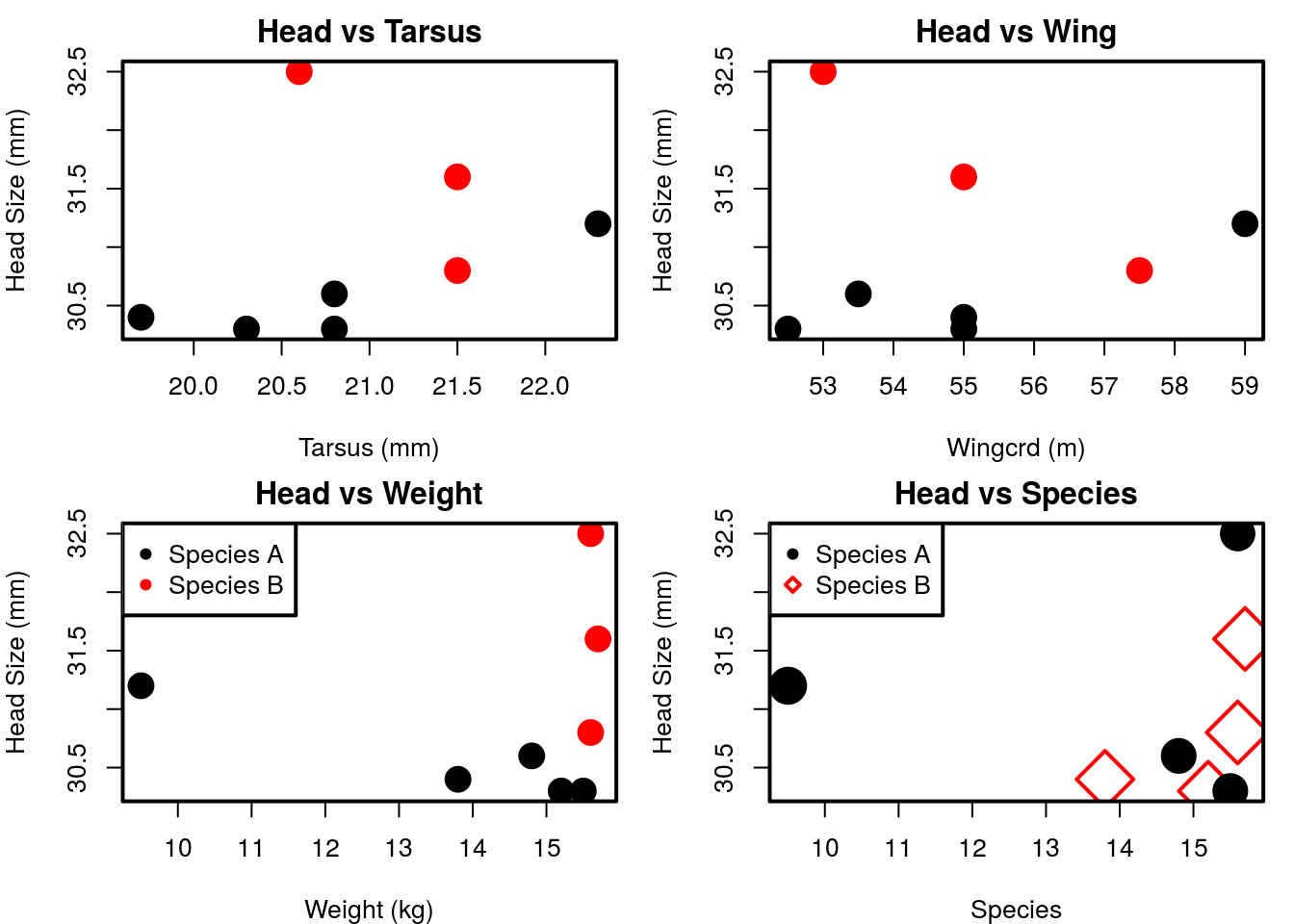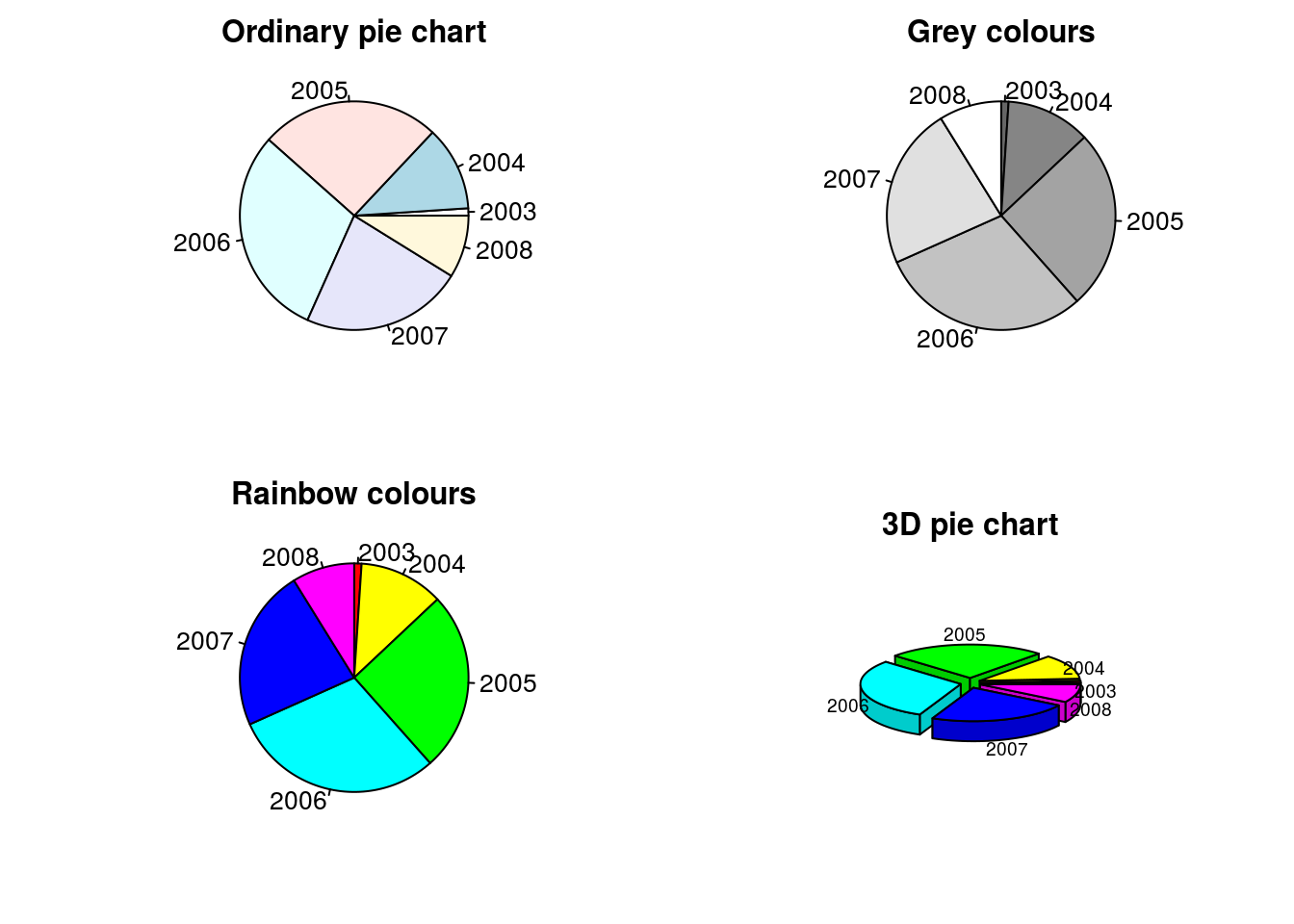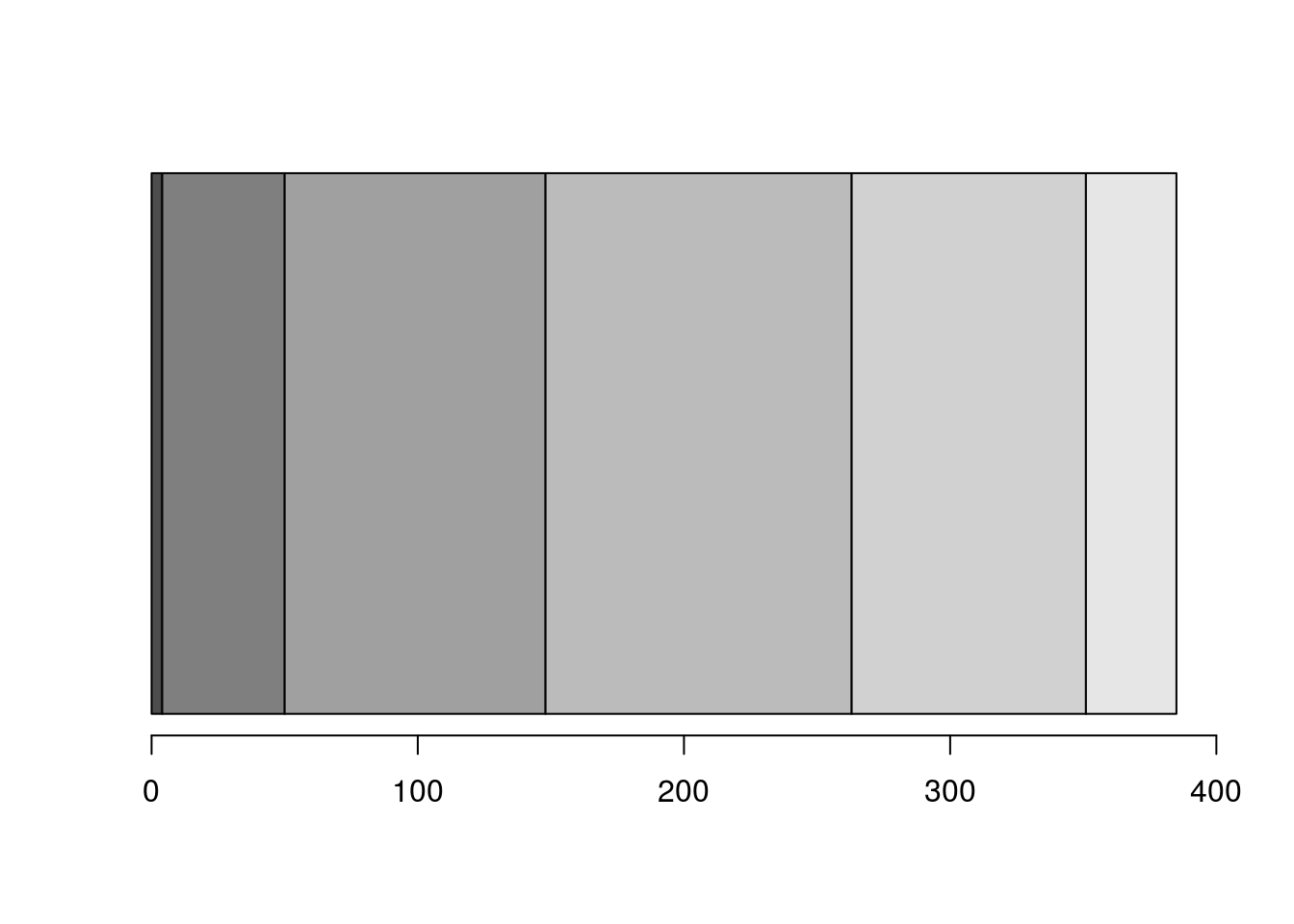# Bestiary of Graphics

Here, we illustrate the variety of different graphs possible in R. These are all done with the base R install. Additional packages such as lattice and ggplot2 provide more options and could be looked at once you are familiar with these.

### Examples of best (and worst) practice

Functions: hist(), barplot(), pie(), stripchart(), boxplot(), dotchart(), coplot()

Packages: vioplot, plotrix

R code: r code .txt

# Learning Goals

A. Foundational Knowledge

• Modify default plots using the graphical parameters function

B. Application

• Make plots according to specific spublisher or thesis requirements

C. Integration & Human Dimension

• Understand the presentation of data
• Consider how best to present your data to tell its story

## Data

Bird data.

``````BirdData <- data.frame(
Tarsus  = c(22.3, 19.7, 20.8, 20.3, 20.8, 21.5, 20.6, 21.5),
Head    = c(31.2, 30.4, 30.6, 30.3, 30.3, 30.8, 32.5, 31.6),
Weight  = c(9.5, 13.8, 14.8, 15.2, 15.5, 15.6, 15.6, 15.7),
Wingcrd = c(59, 55, 53.5, 55, 52.5, 57.5, 53, 55),
Species = c('A', 'A', 'A', 'A', 'A',  'B', 'B', 'B')
)``````

# Graph Selection

Choosing the most appropriate graph for the data is one of the most important decisions you can make in presenting your results.

Here, we will look at some basic graphs, based on whether the x (predictor/independent variable) and y (response/dependent variable) are categorical or continuous:

1. Single continuous

2. Predictor and response are continuous

3. Single categorical

4. Categorical predictor and continous response

Stephen Few has a great Graph Selection Matrix

# The Bestiary

For each graph type, we will look at the default appearance and some of the more useful arguments we can alter.

# A single continuous variable, the histogram: hist()

**data:** single continuous variable

use: illustrating counts or distributions of data

example: bird data

Histograms present the frequency (count) or probability density distribution of a vector of data.

• `breaks` describes the number of bins or breakpoints between bins.
• `freq = TRUE` defaults to counts; set to `FALSE` to plot probability distribution.
• `plot = TRUE` default will plot the histogram. Set to `FALSE` to not plot, and store the counts in an object for future use.
``````# set layout and margins
par(mfrow = c(2, 2), mar = c(3, 3, 2, 1), lwd = 2)

hist(BirdData\$Head, breaks = 10, main = "breaks = 10")
hist(BirdData\$Head, breaks = seq(from = 30, to = 33, by = 0.25), main = "breaks = seq()" )

# plot probability density distribution
hist(BirdData\$Head, breaks = seq(from = 30, to = 33, by = 0.25), freq = FALSE, main = "probability density" )``````Fig. Some histograms

# Two continuous variables, predictor and response, the scatterplot / x-y plot: plot()

**data:** continuous response; continuous predictor.

use: illustrating relationships and correlations between continuous variables, …

example: BirdData

Here, however, we add a legend to two of the panels, using `legend()`.

``````par(mfrow = c(2, 2), mar = c(4, 4, 2, 1), lwd = 2)

plot(Head ~ Tarsus, data = BirdData,
xlab = 'Tarsus (mm)',
pch = 20,
col = Species,
cex = 3)

plot(Head ~ Wingcrd, data = BirdData,
xlab = 'Wingcrd (m)',
pch = 20,
col = Species,
cex = 3)

plot(Head ~ Weight, data = BirdData,
xlab = 'Weight (kg)',
pch = 20,
col = Species,
cex = 3)
# Here, we add a legend to this plot
legend('topleft',
pch = c(20, 20), col = c(1, 2),
legend = c('Species A', 'Species B')
)

plot(Head ~ Weight, data = BirdData,
xlab = 'Species',
pch = c(20, 23),
col = 1:2,
cex = Tarsus/5)
# Another legend
legend('topleft',
pch = c(20, 23), col = c(1, 2),
legend = c('Species A', 'Species B')
)``````Fig. Some scatterplots

# Categorical predictor/independent variable

## The pie chart: pie()

A pie chart is a circular representation of counts or proportion, with the angle corresponding to the value of each category.

data: usually counts or proportion response; categorical predictor

use: never! (unless it involves real pie)

example: BirdFlu

We have annual data from the years 2003-2008. Has the total number of bird flu cases has increased over time?

Load the data and select only cases columns.

``````BFdata <- read.table(file = 'http://www.simonqueenborough.info/R/basic/data/birdflu_corrected.txt', header = TRUE, sep = '\t')
# select cases columns only
BFcases <- BFdata[, c('cases03', 'cases04', 'cases05', 'cases06', 'cases07', 'cases08')]
names(BFdata)``````
``````##   "Country"  "cases03"  "deaths03" "cases04"  "deaths04" "cases05"
##   "deaths05" "cases06"  "deaths06" "cases07"  "deaths07" "cases08"
##  "deaths08"``````
``str(BFdata)``
``````## 'data.frame':    15 obs. of  13 variables:
##  \$ Country : Factor w/ 15 levels "Azerbaijan","Bangladesh",..: 1 2 3 4 5 6 7 8 9 10 ...
##  \$ cases03 : int  0 0 0 1 0 0 0 0 0 0 ...
##  \$ deaths03: int  0 0 0 1 0 0 0 0 0 0 ...
##  \$ cases04 : int  0 0 0 0 0 0 0 0 0 0 ...
##  \$ deaths04: int  0 0 0 0 0 0 0 0 0 0 ...
##  \$ cases05 : int  0 0 4 8 0 0 20 0 0 0 ...
##  \$ deaths05: int  0 0 4 5 0 0 13 0 0 0 ...
##  \$ cases06 : int  8 0 2 13 1 18 55 3 0 0 ...
##  \$ deaths06: int  5 0 2 8 0 10 45 2 0 0 ...
##  \$ cases07 : int  0 0 1 5 0 25 42 0 2 1 ...
##  \$ deaths07: int  0 0 1 3 0 9 37 0 2 0 ...
##  \$ cases08 : int  0 1 0 3 0 7 18 0 0 0 ...
##  \$ deaths08: int  0 0 0 3 0 3 15 0 0 0 ...``````

Now we make a vector of the sum of each year’s cases using `colSums()`, and add names to each element using `names()`.

``````Cases <- colSums(BFcases)
names(Cases) <- 2003:2008
Cases``````
``````## 2003 2004 2005 2006 2007 2008
##    4   46   98  115   88   34``````

The `pie()` function requires a vector of non-negative integers. The names associated with each element are added as labels.

The default direction of `pie()` is anti-clockwise. This can be reversed setting `clockwise = TRUE` (the second pie chart).

For those who will sink so low, there is also a 3-D pie chart function in the ‘plotrix’ package…

``````par(mfrow = c(2, 2), mar = c(3, 3, 2, 1))
pie(Cases, main = "Ordinary pie chart")
pie(Cases, col = gray(seq(0.4, 1.0, length = 6)), clockwise = TRUE, main = "Grey colours")
pie(Cases, col = rainbow(6), clockwise = TRUE, main = "Rainbow colours")
#install.packages('plotrix')
library(plotrix)
pie3D(Cases, labels = names(Cases), explode = 0.1, main = "3D pie chart", labelcex = 0.6) ``````Fig. Some pie charts

#### A word of caution

Most professional statistical graphicicians recommend against using pie charts for the following reasons:

1. Values of all categories need to sum to a meaningful whole
2. Categories need to be mutually exclusive
3. Category ‘other’ may hide important information
4. It is hard for the human eye to measure angles, and therefore the size of each slice
5. Pie charts often require extra labels and legends to interpret (low data:ink ratio)

See here for a greater discussion of these issues.

## The stacked bar plot (better than a pie chart)

An improvement on the pie chart is the stacked bar plot. This allows the viewer to more readily compare the sizes of each section because we also have an axis. But.. there are no labels (although we could add them with `text()` or `legend()`).

``````# To get the 'stacked' bars, we need to convert our vector to a matrix (barplot() will only stack a matrix).
barplot(as.matrix(Cases), horiz = TRUE, xlim = c(0, 400))``````An improvement to the pie chart, the stacked bar plot

The `barplot()` function is discussed in more detail below.

# Categorical predictor/independent variable and continous response variable

There are a variety of ways to plot continuous data as a function of categorical predictors.

## If your response data are counts, use a bar chart: barplot()

**data:** continuous or count response; categorical or binned continuous predictor

use: comparing categories, showing distributions, …

example: BirdFluDeath

We use the bird flu data again, but this time select the deaths columns.

``````BFdata <- read.table(file = 'http://www.simonqueenborough.info/R/basic/data/birdflu_corrected.txt', header = TRUE, sep = '\t')
# select cases columns only
BFdeaths <- BFdata[, c('deaths03', 'deaths04', 'deaths05', 'deaths06', 'deaths07', 'deaths08')]
names(BFdeaths)``````
``##  "deaths03" "deaths04" "deaths05" "deaths06" "deaths07" "deaths08"``
``str(BFdeaths)``
``````## 'data.frame':    15 obs. of  6 variables:
##  \$ deaths03: int  0 0 0 1 0 0 0 0 0 0 ...
##  \$ deaths04: int  0 0 0 0 0 0 0 0 0 0 ...
##  \$ deaths05: int  0 0 4 5 0 0 13 0 0 0 ...
##  \$ deaths06: int  5 0 2 8 0 10 45 2 0 0 ...
##  \$ deaths07: int  0 0 1 3 0 9 37 0 2 0 ...
##  \$ deaths08: int  0 0 0 3 0 3 15 0 0 0 ...``````
``````# Make a vector as before
Deaths <- colSums(BFdeaths)
names(Deaths) <- 2003:2008
Deaths``````
``````## 2003 2004 2005 2006 2007 2008
##    4   32   43   79   59   26``````

How have the number of cases and deaths changed over time?

``````par(mfrow = c(2, 2), mar = c(3, 3, 2, 1), lwd = 2)
barplot(Cases, main = "default barplot of Cases")

# Create a new variable
Counts <- cbind(Cases, Deaths) # makes a matrix
barplot(Counts, main = "default with 2-column matrix" )
barplot(t(Counts), col = gray(c(0.5, 1)), main = "stacked")
barplot(t(Counts), beside = TRUE, main = "beside") ``````